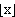# floor function

The floor function of x, historically called the greatest integer function, is the greatest integer less than or equal to x.  This function is sometimes written [x], but is best written(a notation that was suggested by Iverson in 1962) to differentiate it from the ceiling function.

Examples: [3.14159]=3, [-3.14159]=-4, and [log(n)/log(10)]+1 is the number of digits in the decimal expansion of the positive integer n.